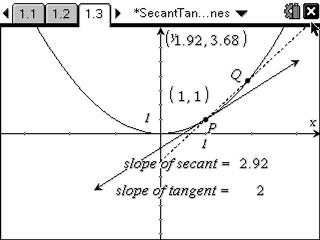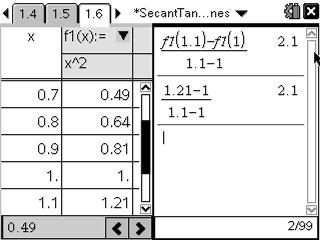# Activities

••• ##### Subject Area

• Math: Calculus: Derivatives

• ##### Author9-12

15 Minutes

• ##### Device
• TI-Nspire™ Navigator™
• TI-Nspire™
• TI-Nspire™ CAS
• ##### Software

TI-Nspire™
TI-Nspire™ CAS

2.0

## Secant and Tangent Lines#### Activity Overview

In this activity, students will observe the slopes of the secant line and tangent line as point Q on the function approaches the other point P. They will also determine the average rate of change for an interval and approximate the instantaneous rate of change using the slope of the secant line.

#### Key Steps

•In this activity, students will explore secant and tangent lines of the graph y = x2 visually by grabbing point Q and moving it toward point P. They are asked to make an observation about the lines and the values of the slopes.

•Students are asked to determine the value of the average rate of change of f1(x) on the interval [1, 1.1] using the graph and table of values. They will then write and calculate an expression to estimate the instantaneous rate of change of f1(x) at x = 1.